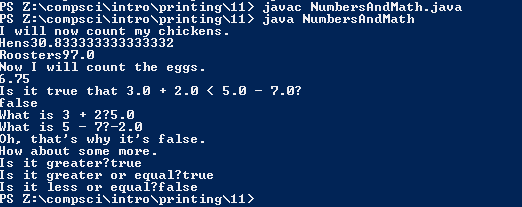# Assignemnt #11 - Numbers And Math

## Code

```    /// Name: Caelan Dorstad
/// Period: 6
/// Program Name: NumbersAndMath
/// File Name: NumbersAndMath.java
/// Date Finished: 9/20/2015

public class NumbersAndMath{
public static void main(String[]args){

System.out.println("I will now count my chickens.");

System.out.println("Hens" + (25.0 + 35.0 / 6.0) ); // 35 divide by 6 plus 25
System.out.println("Roosters" + (100.0 - 25.0 * 3.0 % 4.0) ); // 75 divide by 4 = 18...3 so 100 - 3 = 97

System.out.println("Now I will count the eggs.");

System.out.println(3.0 + 2.0 + 1.0 - 5.0 + 4.0 % 2.0 - 1.0 / 4.0 + 6.0); // 4.0 % 2.0 = 0, 1.0 / 4.0 = 0.25 3.0 + 2.0 + 1.0 - 5.0 + 0 - 0.25 + 6 = 6.75

System.out.println("Is it true that 3.0 + 2.0 < 5.0 - 7.0?" );

System.out.println(3.0 + 2.0 < 5.0 - 7.0); // 5 is greater than -2

System.out.println("What is 3 + 2?" + (3.0+2.0)); // 3 + 2 = 5
System.out.println("What is 5 - 7?" + (5.0-7.0)); // 5 - 7 = -2

System.out.println("Oh, that's why it's false.");